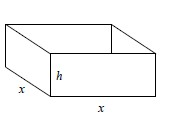### Home > A2C > Chapter 14 > Lesson 14.9.1.2 > Problem9-28

9-28.

An open box is constructed as shown at right. The sides of the box are made out of sheet metal costing $1$ per square foot while the bottom is made of a more expensive metal costing $3$ per square foot. How much, in terms of x and h, will it cost to construct the box?

1. Find the surface area.
2. Sum up the cost of each part.

Surface Area $= x^2 + 2xh + 2xh = x^2 + 4xh$

Cost of Construction $= (3\text{ dollars})x^2 + (1\text{ dollar}) 4xh = 3x^2 + 4xh$